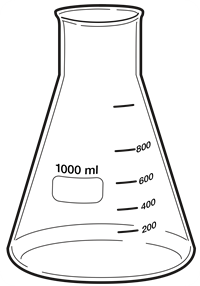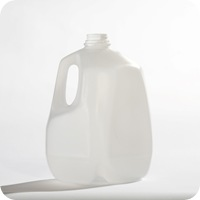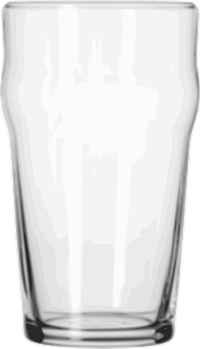# How many milliliters in a liter (gallon, quart, ounce, gram, centimeter, cup or pint)

How many milliliters in a liter? 1000 milliliters in a liter

How many grams in an ounce (pound, pound, kilogram, cup, teaspoon, quarter)

How many liters in a gallon (quart, milliliter, cup, pint, ounce)

How many cups in a gallon (quart, pint, liter, pound, ounce, oz)

Liter has always been a common measurement unit for measuring liquids. You probably heard that adults need to drink at least 2 liters of water every day. The soda bottles are labeled as 1 L. It can also be used to measure the solids that can be poured, like salt. You can say that container has half a liter of salt. The liter is the American spelling. Its symbol is l and L. 1 liter is equal to 1000 cubic centimeters, (1 / 1000) cubic meter or 1 cubic decimeter. 1 liter takes up the space as box which has all the sides to be 10 centimeters.1 cubic centimeter = 1ml

1 cubic centimeter = 0.001 l

So, 1 ml = 0.001 l therefore, 1 liter is 1000 milliliters (1 l = 1000 ml)

How many milliliters in a gallon3785.41 milliliters in a gallon

946.353 milliliters in a quart

To find out how many liters in gallons, multiply by the factor or use the converter below.

1 Gallon (liquid, US) is 3.7854118 L

1 Gallon (Dry, US) = 4.4048838 L

1 Gallon (British) = 4.54609 L

For fuel economy conversions such as mpg to liters/100km, there is another value. Gallon is a very popular measurement unit in both the British and US calculations. In US mathematical operations, there are two types of gallons: 1. Dry 2. Liquid and in the British system, there is only one type of gallon. It is usually used in daily life. The dry gallon is mostly used in calculating the wheat, corn and other types of dry goods from quantifying the wine and beer to fuel consumption (measuring the fuel efficiency as miles per gallon). Subsequently the metric system is acknowledged, this measurement unit gallon has been mostly substituted by liter. The abbreviation is “gal”.

Using the above information, we can find out how many milliliters are there.

1 gal = 3.7854118 L, 3.7854118 L = 3785.41 ml

1 quart = 0.946353, move the decimal place three times in th right side because there are three zeros in thousand. 1 quart = 946.353 ml.

How many milliliters in an ounce29.5735 milliliters in an ounce

1 milliliter in a gram

1 milliliter in a cubic centimeter

236.588 milliliters are in a cup

473.176 milliliters in a pint

2000 milliliters are in 2 liters

We know a cups holds 8 ounce, 8 ounce means 240 ml. To find how much is in each ounce, divide 240 ml by 8 ounce. You will get 30. The exact value is 29.5735 and the mathematical value is 30.

In mathematical terms, (240 / 8) = 30.

Calculating grams to milliliters is confusing for people because gram is common for solids. 1 ml of water weighs one gram in typical situations, including for cooking recipes and math and science problems (unless another stated). There is no need to do any math: the measurement in milliliters and grams are at all times identical such as 3 gram is equal to 3 ml.

3 g = 3ml

This site uses Akismet to reduce spam. Learn how your comment data is processed.